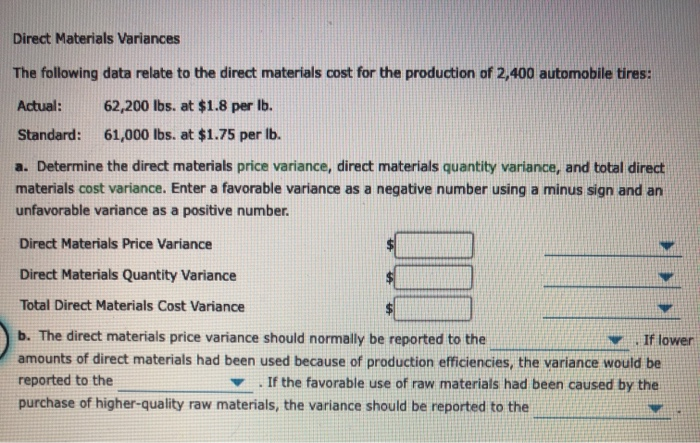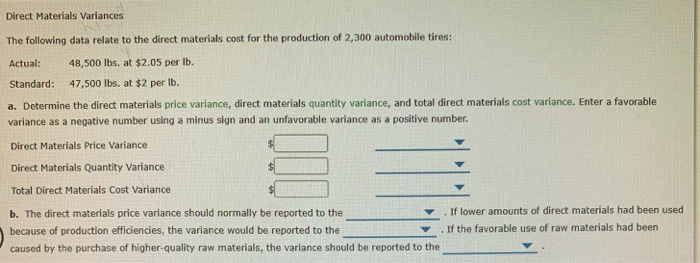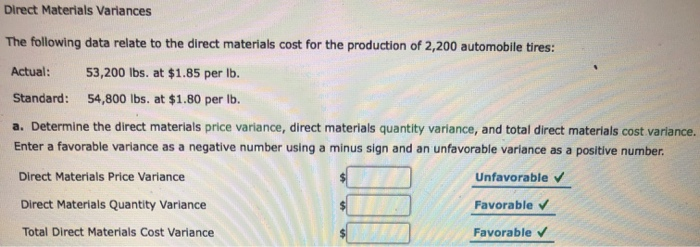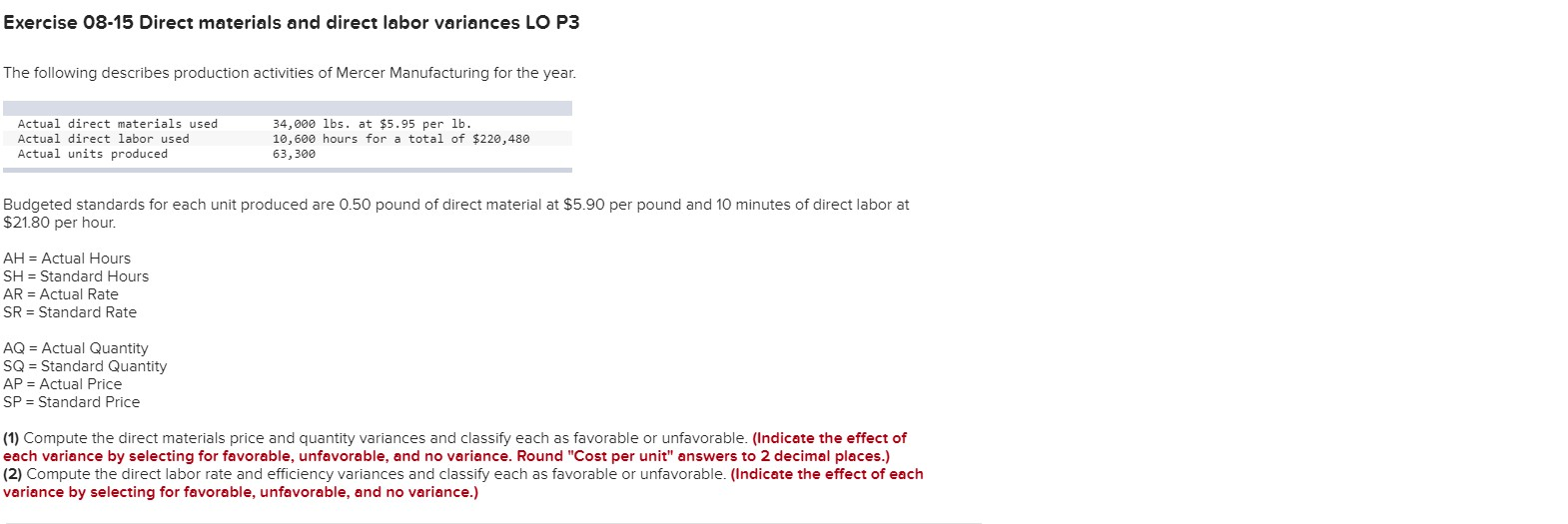Question

Direct Materials Variances

The following data relate to the direct materials cost for the production of 2,400 automobile tires:

 Actual: 61,700 lb. at \$2.05 Standard: 62,900 lb. at \$2.00

a. Determine the direct materials price variance, direct materials quantity variance, and total direct materials cost variance. Enter a favorable variance as a negative number using a minus sign and an unfavorable variance as a positive number.

 Price variance \$ Unfavorable Quantity variance \$ Favorable Total direct materials cost variance \$ Unfavorable

b. The direct materials price variance should normally be reported to the Purchasing Department . If lower amounts of direct materials had been used because of production efficiencies, the variance would be reported to the Production Supervisor . If the favorable use of raw materials had been caused by the purchase of higher-quality raw materials, the variance should be reported to the Purchasing Department .

• Requirement ‘a’
 Material Price Variance ( Standard Rate - Actual Rate ) x Actual Quantity ( \$                        2.00 - \$                       2.05 ) x 61700 -3085 Variance \$              3,085.00 Unfavourable-U

 Material Quantity Variance ( Standard Quantity - Actual Quantity ) x Standard Rate ( 62900 - 61700 ) x \$                           2.00 2400 Variance \$              2,400.00 Favourable-F

Total Direct Material Cost Variance = 3085 Unfavourable + 2400 favourable = \$ 685 Unfavourable,
OR

 Total Direct Material Cost Variance ( Standard Cost - Actual Cost ) ( \$           125,800.00 - \$          126,485.00 ) -685 Variance \$                  685.00 Unfavourable-U

• Requirement ‘b’. Complete and Correct.

#### Earn Coins

Coins can be redeemed for fabulous gifts.

Similar Homework Help Questions
• ### Direct Materials Variances The following data relate to the direct materials cost for the production of...Direct Materials Variances The following data relate to the direct materials cost for the production of 2,400 automobile tires: Actual: 62,200 lbs. at \$1.8 per lb. Standard: 61,000 lbs. at \$1.75 per lb. a. Determine the direct materials price variance, direct materials quantity variance, and total direct materials cost variance. Enter a favorable variance as a negative number using a minus sign and an unfavorable variance as a positive number. Direct Materials Price Variance Direct Materials Quantity Variance Total Direct...

• ### Direct Materials Variances The following data relate to the direct materials cost for the production of...

Direct Materials Variances The following data relate to the direct materials cost for the production of 50,000 automobile tires: Actual: 725,000 lbs. at \$3.00 per lb. Standard: 730,000 lbs. at \$2.95 per lb. a. Determine the direct materials price variance, direct materials quantity variance, and total direct materials cost variance. Enter a favorable variance as a negative number using a minus sign and an unfavorable variance as a positive number. Direct Materials Price Variance \$ Direct Materials Quantity Variance \$...

• ### Direct Materials Variances The following data relate to the direct materials cost for the production of 2,300 autom...Direct Materials Variances The following data relate to the direct materials cost for the production of 2,300 automobile tires: Actual: 48,500 lbs. at \$2.05 per Ib. Standard: 47,500 lbs. at \$2 per lb. a. Determine the direct materials price variance, direct materials quantity variance, and total direct materials cost variance. Enter a favorable variance as a negative number using a minus sign and an unfavorable variance as a positive number. Direct Materials Price Variance Direct Materials Quantity Variance Total Direct...

• ### Direct Materials Variances The following data relate to the direct materials cost for the production of 2,100 automobile...

Direct Materials Variances The following data relate to the direct materials cost for the production of 2,100 automobile tires: Actual: 58,000 lbs. at \$1.75 per lb. Standard: 59,200 lbs. at \$1.70 per lb. a. Determine the direct materials price variance, direct materials quantity variance, and total direct materials cost variance. Enter a favorable variance as a negative number using a minus sign and an unfavorable variance as a positive number. Direct Materials Price Variance \$ Direct Materials Quantity Variance \$...

• ### Direct Materials Variances The following data relate to the direct materials cost for the production of 50,000 automobil...

Direct Materials Variances The following data relate to the direct materials cost for the production of 50,000 automobile tires: Actual: 725,000 lbs. at \$3.00 per lb. Standard: 730,000 lbs. at \$2.95 per lb. a. Determine the direct materials price variance, direct materials quantity variance, and total direct materials cost variance. Enter a favorable variance as a negative number using a minus sign and an unfavorable variance as a positive number. Direct Materials Price Variance \$ Direct Materials Quantity Variance \$...

• ### Direct Materials Variances The following data relate to the direct materials cost for the production of...Direct Materials Variances The following data relate to the direct materials cost for the production of 2,200 automobile tires: Actual: 53,200 lbs. at \$1.85 per lb. Standard: 54,800 lbs. at \$1.80 per lb. a. Determine the direct materials price variance, direct materials quantity variance, and total direct materials cost variance. Enter a favorable variance as a negative number using a minus sign and an unfavorable variance as a positive number Direct Materials Price Variance Unfavorable Favorable Direct Materials Quantity Variance...

• ### Direct Materials Variances The following data relate to the direct materials cost for the production of...

Direct Materials Variances The following data relate to the direct materials cost for the production of 2,500 automobile tires: Actual: 53,500 lbs. at \$1.85 per lb. Standard: 55,100 lbs. at \$1.8 per lb. a. Determine the direct materials price variance, direct materials quantity variance, and total direct materials cost variance. Enter a favorable variance as a negative number using a minus sign and an unfavorable variance as a positive number. Direct Materials Price Variance \$ 2,675 Unfavorable Direct Materials Quantity...

• ### The following data relate to direct materials costs for February: Materials cost per yard: standard, \$1.93;...

The following data relate to direct materials costs for February: Materials cost per yard: standard, \$1.93; actual, \$2.05 Yards per unit: standard, 4.63 yards; actual, 5.07 yards Units of production: 9,300 The direct materials quantity variance is a. \$7,897.56 favorable b. \$7,897.56 unfavorable c. \$8,388.60 unfavorable d. \$8,388.60 favorable

• ### Exercise 08-15 Direct materials and direct labor variances LO P3 The following describes production activities of...Exercise 08-15 Direct materials and direct labor variances LO P3 The following describes production activities of Mercer Manufacturing for the year. 34,000 lbs. at \$5.95 per lb 10,600 hours for a total of \$220,480 Actual direct materials used Actual direct labor used Actual units produced 63,300 Budgeted standards for each unit produced are 0.50 pound of direct material at \$5.90 per pound and 10 minutes of direct labor at \$21.80 per hour. AH Actual Hours SH Standard Hours AR Actual...

• ### Direct materials variances

The following data relate to the direct materials cost for the production of 2,000 automobile tires:actual 54600 lbs at 1.80 \$ 98280standard 53400 lbs at 1.85 \$ 98790a. Determine the direct materials price variance, direct materials quantity variance, and total direct materials cost variance. Use the minus sign to enter favorablevariances as negative numbers.Price variance: _______________Quantity variance: _____________Total direct materials cost variance ____________thank u